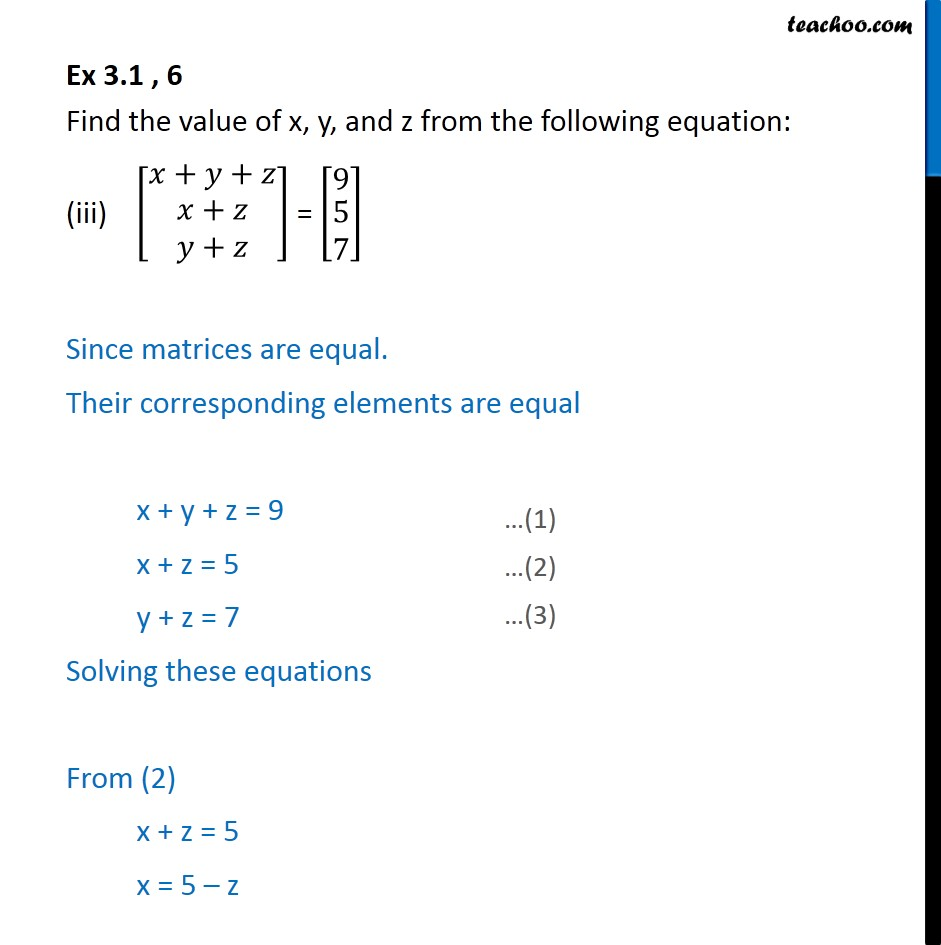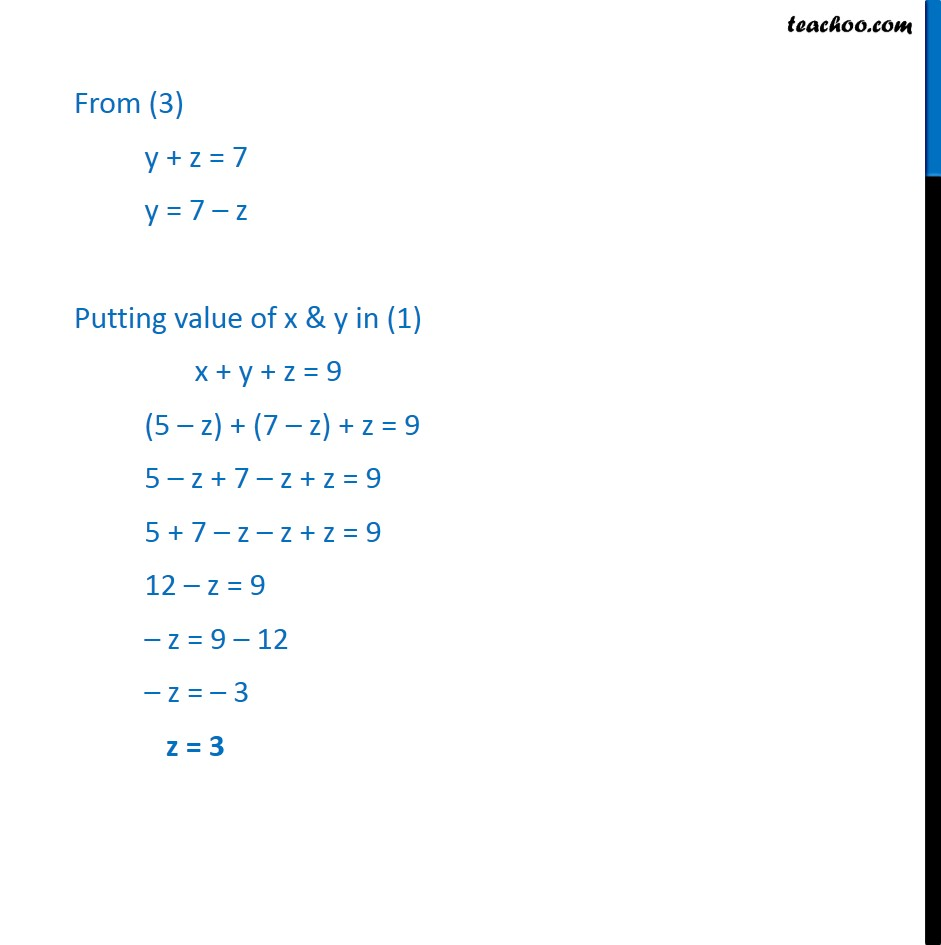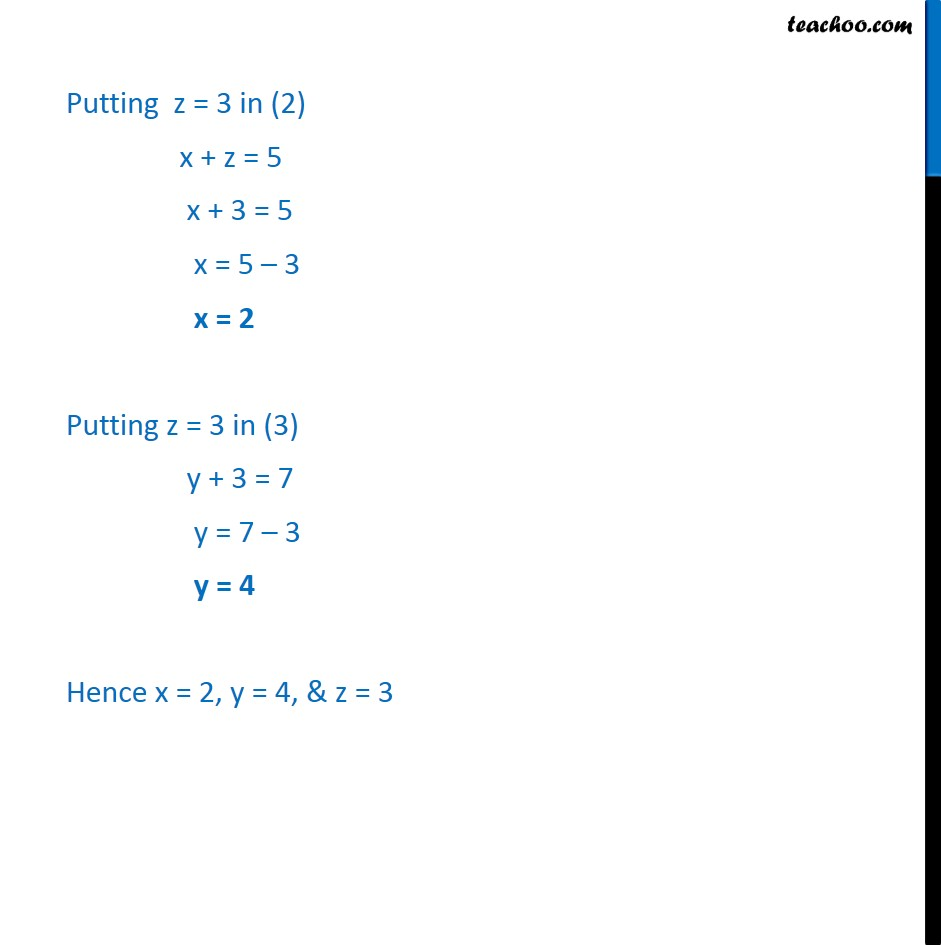Ex 3.1

Chapter 3 Class 12 Matrices
Serial order wiseLearn in your speed, with individual attention - Teachoo Maths 1-on-1 Class

### Transcript

Ex 3.1 , 6 Find the value of x, y, and z from the following equation: (iii) [ 8( + + @ ( + @ + ))] = [ 8(9@ ([email protected]))] Since matrices are equal. Their corresponding elements are equal x + y + z = 9 x + z = 5 y + z = 7 Solving these equations From (2) x + z = 5 x = 5 z From (3) y + z = 7 y = 7 z Putting value of x & y in (1) x + y + z = 9 (5 z) + (7 z) + z = 9 5 z + 7 z + z = 9 5 + 7 z z + z = 9 12 z = 9 z = 9 12 z = 3 z = 3 Putting z = 3 in (2) x + z = 5 x + 3 = 5 x = 5 3 x = 2 Putting z = 3 in (3) y + 3 = 7 y = 7 3 y = 4 Hence x = 2, y = 4, & z = 3